## Harmonic Logarithm

For all Integersand Nonnegative Integers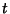, the harmonic logarithms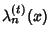of orderand degreeare defined as the unique functions satisfying

1.,

2.has no constant term except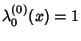,

3.,
where the Roman Symbol''is defined by(1)

(Roman 1992). This gives the special cases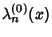(2)(3)

whereis a Harmonic Number(4)

The harmonic logarithm has the Integral(5)

The harmonic logarithm can be written(6)

whereis the Differential Operator, (so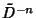is theth Integral). Rearranging gives(7)

This formulation gives an analog of the Binomial Theorem called the Logarithmic Binomial Formula. Another expression for the harmonic logarithm is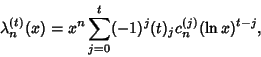(8)

whereis a Pochhammer Symbol andis a two-index Harmonic Number (Roman 1992).

Loeb, D. and Rota, G.-C. Formal Power Series of Logarithmic Type.'' Advances Math. 75, 1-118, 1989.
Roman, S. The Logarithmic Binomial Formula.'' Amer. Math. Monthly 99, 641-648, 1992.